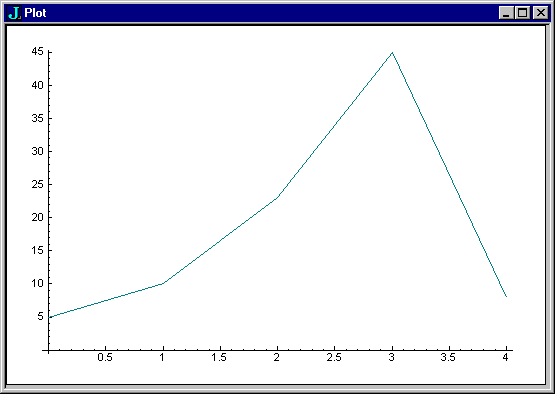>>  <<  Usr  Pri  JfC  LJ  Phr  Dic  Voc  !:  Help  Primer

# Plot

To use the plot facility you need to load it.
```   load 'plot'
```
Try a simple plot.
`   plot 5 10 23 45 8`The plot is in a separate window. Close the plot window to get rid of it.

Now that you can plot data, let's take a look at some of the primitives you were using in the previous section.
```   plot 2 ^ i. 5
plot ? 5 \$ 100
plot ? 50 \$ 100
plot ? 100 \$ 100
plot ? 1 + i. 50
plot ? 1 + i. 100```
A left argument customizes the plot.
`   'TITLE myplot;TYPE bar' plot 2 ^ i. 5`
Or try the sine values you calculated earlier.
`   plot 1 o. i. 16  `
There is a family of utilities defined in script trig.ijs you could make use of here. Use load to load that script.
```   load 'trig'
plot sin i. 16```
To produce a finer plot you need to provide more results over a similar range.
```   plot sin 0.2 * i.60
plot cos 0.2 * i.60
```

>>  <<  Usr  Pri  JfC  LJ  Phr  Dic  Voc  !:  Help  Primer